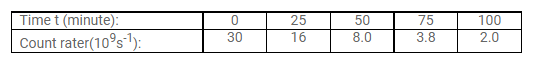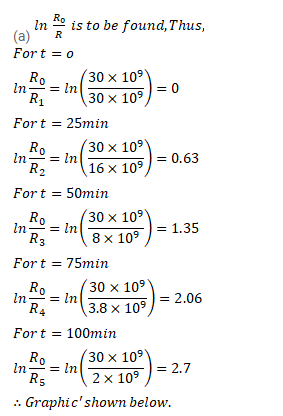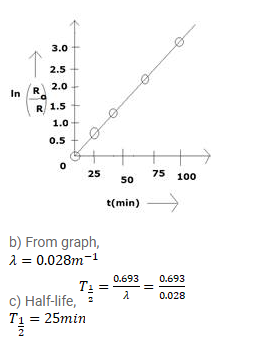# The count rate of nuclear radiation coming from a radioactive sample containing

Question:

The count rate of nuclear radiation coming from a radioactive sample containing ${ }^{128}$ I varies with time as follows.(a) Plot In ( $R_{0} / R$ ) against t.

(b) from the slope of the best straight line through the points, find the decay constant $\lambda$.

(c) Calculate the half-life $t_{1 / 2}$.

Solution: